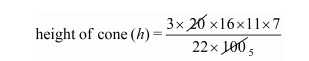# A rectangular vessel of dimensions 20 cm × 16 cm × 11 cmQuestion:

A rectangular vessel of dimensions 20 cm × 16 cm × 11 cm is full of water. This water is poured into a conical vessel. The top of the conical vessel has its radius 10 cm. If the conical vessel is filled completely, determine its height.

Solution:

Volume of cone $=\frac{1}{3} \pi \mathrm{r}^{2} \mathrm{~h}$

$\frac{1}{3} \pi \mathrm{r}^{2} \mathrm{~h}=20 \times 16 \times 11$$=33.6 \mathrm{~cm}$